# Torque Pro on the Kona - overview and setup for interested owners

Discussion in 'Hyundai Kona Electric' started by KiwiME, Sep 26, 2019.

1. Pid 220101 what should use instead?

2. Thinking about that, what you've calculated is the portion of the "90% capacity retention" cycles that you have "used up", rather than the absolute SoH that a Leaf owner might quote.

I would suggest that it's actually ((100% - 2%) x 10%)) + 90% which would put your SoH at 99.8%.

3. This works on Android 9 so will work on Android 10. It works with the car turned on, maybe not with it off.
Note that the data is converted correctly, but the label underneath is NOT automatically changed. To change the label, delete the dashboard gauge then reload it.

4. I’ll take that back, while it appears to work on any Android version, it doesn’t do what you would expect, which is a straight conversion.
Instead it treats the raw numeral as an F value (i.e. 23F not 23C), then converts that to C, so 23C gets converted to -5C!! Not useful...

5. Thank You!

6. I was trying to answer a question elsewhere about the meaning of SoC so I opened up some old data to find something relevant. In doing so I thought this was an interesting graph to demonstrate how well SoC tracks battery energy delivery.

The SoC is not based directly on battery voltage or some other parameter that can be measured at any instant. In fact, as I discussed elsewhere in this thread, it's based primarily on energy accounting. The BMS rarely knows exactly what's in the 'bank' right now but it knows exactly how much is going in and how much is going out whenever the BMS is active, technically known as coulomb counting. However, if it never had a way of calibrating it's position relative to the actual SoC once in a while it could drift off with accumulating errors. So, it needs to correct itself occasionally, how often so depends on how accurately it can track usage.

If you charge towards 100% it knows it's fully charged by when the charging current drops to near zero on the constant-voltage portion of the charge curve. It can also check the open-circuit voltage at startup or shutdown, that is, while the BMS is powered-off the 12V battery, before the main contactor closes and disturbs the traction battery.

This trip data was taken last July in our winter, but that means perhaps 12-18°C. But it doesn't really matter what the driving conditions were because we're only looking at the traction batteries activities. The resulting use and efficiency of the energy provided is immaterial to this graph. FWIW, the round trip length was about 300 km over about 3.5 hours.

If you first follow the red line starting from 100% SoC at the top-left, this is energy going out of the battery (CED) with the accumulated energy on the X-axis as SoC diminishes on the Y-axis. If you follow the green line, this is the small amount of energy going back into the battery by way of regen (CEC), again as accumulated energy by diminishing SoC.

Along any horizontal line the blue line is simply the red line kWh value minus the green line kWh value. But the reason I plotted all these values against SoC instead of time or distance is because you'll notice that the blue line is very close to being perfectly linear. There is a black linear (straight) trendline accompanying the blue line for reference but it's nearly invisible.

That's because, in a simplistic way, this is how the SoC is actually calculated as you drive. There will be minor corrections for battery cycle efficiency and temperature that would improve that linearity further.

The slope of the blue line (the trendline actually) is 1.572. It's worth noting that 100/1.572 = 63.6, so it's not unreasonable to consider SoC to be a good percent indicator of what remains out of 64 kWh.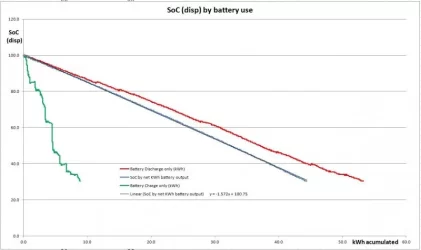electriceddy likes this.
7. I'm currently working on a personal project to use an esp32 (ttgo t-display) to read pids and display them with other calculated values. I'm calculating the net energy used (CED-CED) like you do as well as the net capacity used(Ah) (CDC-CCC). I calculate the expected net energy used for 100% soc and the same for the expected net capacity used. I'm very closed to be able to save the values realtime to a google sheet but can still display the values on the esp32 while driving.
I did 3 drives from 100% to 5-8% soc in the past 3 weeks. The ambiant temperature here is around -5 degC.
My observations is that the net energy used per %soc at higher soc is greater then at lower soc. From 100% to 90%, it used around 7 kWh while from 20% to 10% it used around 5.7 kWh. The expected net energy for a full discharge calculated at 90% was giving me 70 kWh. It finally came down close to 64 kWh close to 5% soc.
On the other hand, the net capacity used was very linear, giving 18 Ah per 10% soc. All through the discharge, the calculated expected Ah for 100% soc remained around 180 Ah.

8. Thinking about this question over the last few days, I don't have any suitable data yet to confirm the lack of correlation of net cumulative energy to SoC at lower SoCs because until now I've ignored the cumulative current values in all data I've collected. Yesterday I logged the data over a limited mid-SoC range and it shows (FWIW) that both match approximately. It's true that SoC has been defined as both Ah or energy in the industry literature I've read, however I haven't found any basis for why one or the other would be preferable. But, it must be accepted in principle that it's the product of current and voltage that provides the energy to move the car down the road. As pack voltage is reduced with loss of SoC, more current must be provided to maintain the same power output.

Mulling over this quandary, it seems to me that the cumulative current odometers are not affected by battery working losses, but only deterioration. E.g., the max pack capacity in Ah is available irrespective of cycles until the BMS-defined working capacity runs out of reserve and has to accept the onset of deterioration.

If I use a 180 litre tank of water as an analogy, that volume or any portion can be added or removed over and over without any change in the total maximum tank volume. That cumulative volume change would make it a good representative of SoC if energy linearity was not a consideration as the water pressure is lower at lower tank volumes.

On the other hand, considering energy, there are flow losses which means energy is lost whenever water is added or removed from the tank. The cumulative energy odometers capture this because pack voltage sags and rises on discharge and charge, respectively, due to normal battery resistive losses. Those energy odometers of course represent a realtime calculation based on instantaneous (signed) current and pack voltage sampled at some rate (perhaps in milliseconds) and integrated over time and added to each of the two registers as the current direction dictates.

So, if plotted over the batteries (BMS-defined) full-capacity lifetime, the two energy odometer values will diverge over time because those losses accumulate, but in-theory the current odometers won't. (Noting that once deterioration kicks in the divergence of the two cumulative current values could provide a way to quantify deterioration.)

But, if the energy odometers were used to determine SoC, over many cycles they would lose track of the true value and a recalibration would be required, perhaps from open-circuit pack voltage and historical data. Although the raw data would represent the available energy at any time, it would drift off depending on how aggressive the cycling is between calibrations. I've realized this in the past but have assumed that there is more than enough historical data to accomplish this.

So, I'll have to do another 300 km data-collection trip and delve into the SoC values under 25% that I normally avoid. Meanwhile, from yesterday, in the graph below of Net values from 66 to 54% (SoC dsip), the (Ah) orange line's slope is near the 180 Ah value when the SoC(BMS) is used while the (kWh) blue line is near the 64 kWh value when the SoC(displayed) is referenced. That doesn't entirely surprise me because the understood 60Ah per-cell rating may be the base capacity rather that the BMS-defined useful capacity.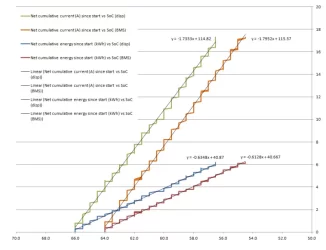herode10 and cyberelectronics like this.
9. ### cyberelectronicsNew Member

@KiwiME I'm using CEC and CED for charge and trip data calc.
What do you think about these CEC/CED (shifting) differences?

1) According to Trip 1 (Charging 47.8kWh) at ODO 4373km = CEC 934.1 - CED 873.6 = 60.5 kWh at SOC disp 77%
=> 60.5 - 49.28 (77%) = 11.2kWh (loss?)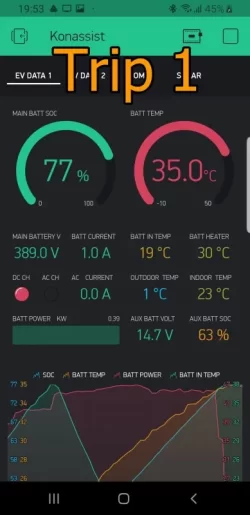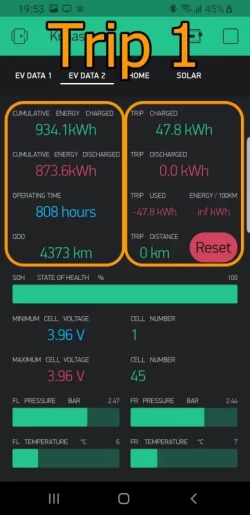(Charge graph + Total Ch 51.4kWh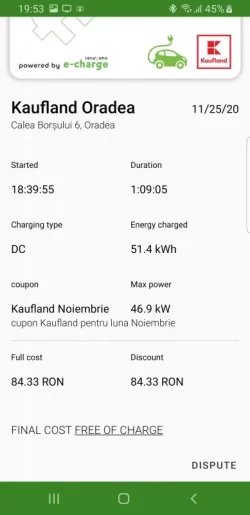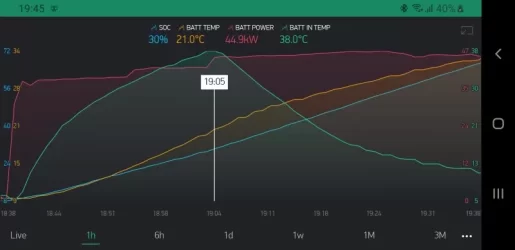)

2) According to Trip 2 at ODO 5991km = CEC 1249.1 - CED 1209.6 = 39.5 kWh at SOC disp 20%
=> 39.5 - 12.8 (20%) = 26.7 kWh (loss?)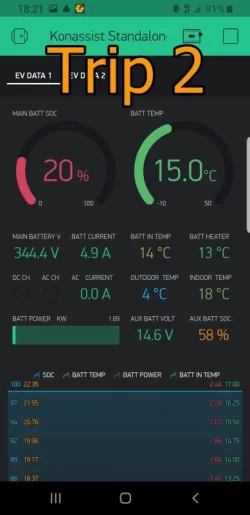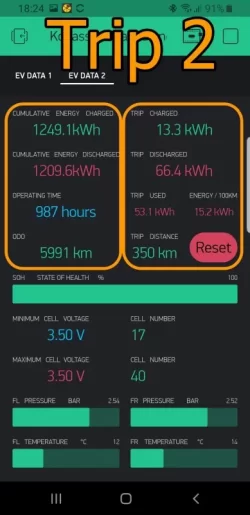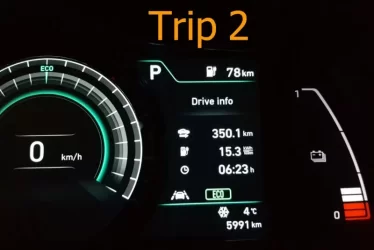Difference (loss?) in 1627km => 26.7-11.2 = 15.5 kWh

Last edited: Jan 11, 2021 at 4:11 AM
10. I don't understand your explanation of what you're trying to achieve but it appears you may have misinterpreting how to use the CEC and CED odometers. You don't use the absolute values to determine the energy use over a trip, only the change in those values, which is why I refer to those as delta CEC and CED.
So, at the trip start record those two values and subtract them from the corresponding values at the end. Then subtract the delta CEC from the delta CED to get the net change in cumulative energy for that trip.
The other reliable and relevant number from the car is the distance driven and so from that you can calculate kWh/100km which totally ignores battery losses much as the car's readouts appear to do, i.e. it's not an overall efficiency (including charging losses) that could be compared with the car's economy rating.

As for SoC, we're discussing what that means and as such it appears so-far that it's a questionable indicator of battery energy level.

11. Very interesting analysis. I like your analogy example.
As I mention, I got more energy spent in the top end of the displayed SOC range (around 80 to 100%) and less in the bottom end. In the middle range, the net energy used is more linear, especially around 40 to 60%. As you mention, energy is function of instantaneous current and voltage. The cell voltage vs SOC is quite linear between 30 to 70% soc but flattens at both ends. The cell capacity for a lithium cell is supposed to remain linear throughout its range. I will be interested to see your results for
Very good analysis and I like your analogy to a tank of water.
I would be interested to a top end SOC net energy used. 66 to 54% is within the linear portion of cell voltage vs soc (30 to 70%). At both end the voltage flattens. Around 50% is the nominal cell voltage were the battery pack energy is calculated: 180Ah x 356v = 64kWh. Thus I would expect a net energy used between 66 to 54% to be very close to 64kWh for such a short trip.

KiwiME likes this.
12. ### cyberelectronicsNew Member

Sorry,
I'm using CEC and CED, exactly as you described.
I'm just thinking about the changing differences (bigger), between CEC and CED values over time (in thousands of kms).

cyberelectronics likes this.
14. As I noted earlier in post #189 the cumulative current odometers are not subject to resistive losses as are the energy odometers. Noting the water tank analogy, the CCC and CDC simply track water going in and out of the 180L tank without caring about what energy was required or recovered to accomplish that. It's been demonstrated by both herode10 and myself that the slope these parameters track in practice (against one or the other SoC values provided by the Kona's BMS) does trend towards 180Ah, as we should expect. That simply means that a linear trendline applied to actual ΔCCC-ΔCDC values does have a rate of change of approximately 180Ah per 100%.

If you consider the water tank initially filled to 50% and then water pumped to and from the tank, the cumulative volume counters would track volume from that level. At any time you could use those two values to understand how far you're off the original 50% fill. But in order to use those relative values as an absolute fill indicator, you need to know that the fill was 50% at the start.

Likewise, in order for the BMS to be able to use the current odometer values, it must also know the initial battery "fill". I knew this was the case back in post #84 when I proposed that the cumulative energy odometers could be used, however I was unsure how this could be obtained.

So, it dawned on me yesterday that as long as the battery still retains full health, the initial SoC is revealed whenever the current odometer values become equal. Below is a plot of CCC and CDC from today's drive and the crossover occurs at 57.4% SoC(displayed). That would have been the SoC when I took the car in for the Campaign 196 update 14 December, noting that all four odometers are zeroed during the update.

Because that initial SoC is also applicable to the energy odometers (CEC and CED) and those values are subject to battery losses. Since being at the initial SoC means there is no error caused by the current charge level, we can derive another useful number - the battery efficiency. So, the second plot is of the cumulative energy odometers where the ratio of those at 57.4% SoC(dis) is 98%.

That means that on average and to-date, 98% of the charging energy was recovered as useful outgoing energy, better than the 96% I had previously estimated. Now, that number could vary between cars and over time, especially if there are more complicated losses happening during high-power DCFC.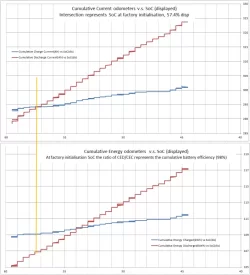herode10 and cyberelectronics like this.